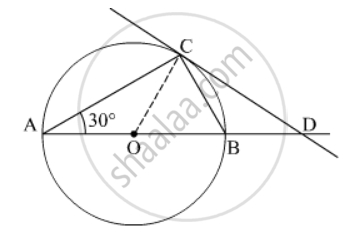Advertisement Remove all ads

# Ab is a Diameter and Ac is a Chord of a Circle with Centre O Such that ∠ B a C = 30 O . the Tangent at C Intersects Ab at a Point D . Prove that Bc = Bd. - Mathematics

Short Note

AB is a diameter  and AC is a chord of a circle with centre such that $\angle BAC = {30}^o$ . The tangent at C intersects AB  at a point D . Prove that BC = BD.

Advertisement Remove all ads

#### SolutionIt is given that ∠BAC = 30° and AB is diameter.

$\frac{AB}{2} = OA = OC \left( \text{Radius} \right)$

ACB = 90°       (Angle formed by the diameter is 90°)

In ∆ABC,

ACB + ∠BAC + ∠ABC = 180°

⇒ 90° + 30° + ∠ABC = 180°

⇒ ∠ABC = 60°

⇒ ∠CBD = 180° – 60° = 120° ( ∠CBD and ∠ABC form a linear pair)

In ∆OCD,

OCD = 90°      (Angle made by Radius on the tangent)

OBC = ∠ABC = 60°

Since OB = OC, ∠OCB = ∠OBC = 60°      (OC = OB = radius)

In ∆OCB,

⇒ ∠COB + ∠OCB + ∠OBC = 180°

⇒ ∠COB + 60° + 60° = 180°

⇒ ∠COB = 60°

In ∆OCD,

COD + ∠OCD  + ∠ODC = 180°

⇒ 60° + 90° + ∠ODC = 90°     (∠COD = ∠COB)

⇒ ∠ODC = 30°

In ∆CBD,

CBD = 120°

BDC = ∠ODC = 30°

⇒ ∠BCD + ∠BDC + ∠CBD = 180°

⇒ ∠BCD + 30° + 120° = 180°

⇒ ∠BCD + 30° = ∠BDC

Angles made by BC and BD on CD are equal, so ∆CBD is an isosceles triangle and therefore, BC = BD.

Is there an error in this question or solution?
Advertisement Remove all ads

#### APPEARS IN

RD Sharma Class 10 Maths
Chapter 8 Circles
Exercise 8.2 | Q 20 | Page 35
Advertisement Remove all ads

#### Video TutorialsVIEW ALL 

Advertisement Remove all ads
Share
Notifications

View all notifications

Forgot password?
Course